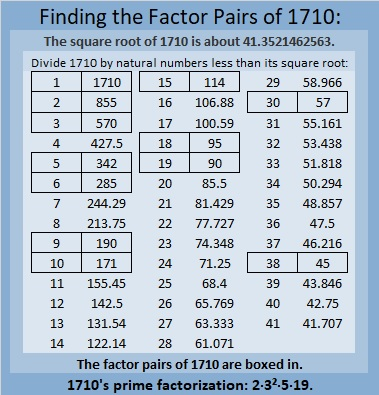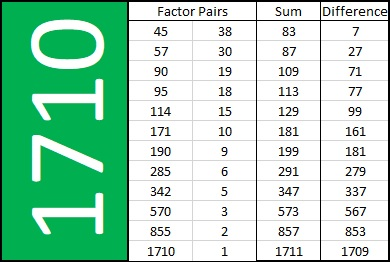# Find the Factor Pairs of 1710. Which One Sums to 181? Which One Subtracts to 181?

### Today’s Puzzle:

1710 has TWELVE factor pairs. Two of those factor pairs are special. If you add up the factors in one of them, you will get 181, but get this, if you subtract the factors in the other one, you will also get 181. Find the two factor pairs that do that, and you will have essentially solved this puzzle:This Sum-Difference puzzle would be helpful if you had to factor any of these trinomials:
45x² – 181x + 38,
95x² – 181x – 18,
90x² + 181x + 19,
38x² + 181x – 45,

The trinomials are in the form ax² + bx + c, where b = 181, and a·c = ±1710. Whether the 1710 is positive or negative makes all the difference in the world when factoring them!

### Factors of 1710:

• 1710 is a composite number.
• Prime factorization: 1710 = 2 × 3 × 3 × 5 × 19, which can be written 1710 = 2 × 3² × 5 × 19.
• 1710 has at least one exponent greater than 1 in its prime factorization so √1710 can be simplified. Taking the factor pair from the factor pair table below with the largest square number factor, we get √1710 = (√9)(√190) = 3√190.
• The exponents in the prime factorization are 1, 2, 1, and 1. Adding one to each exponent and multiplying we get (1 + 1)(2 + 1)(1 + 1)(1 + 1) = 2 × 3 × 2 × 2 = 24. Therefore 1710 has exactly 24 factors.
• The factors of 1710 are outlined with their factor pair partners in the graphic below.The following chart lists 1710’s factor pairs with their sums and differences:### More About the Number 1710:

1710 is the hypotenuse of a Pythagorean triple:
1026-1368-1710, which is (3-4-5) times 342.

# 1390 Find the Factors (ax±b)(cx±d)

I liked making a puzzle using trinomials earlier today. This one will take more skill to solve even though it contains fewer trinomials. Some of the factors will have negative numbers, and the leading coefficients of the trinomials are not 1.

In this puzzle, you can see the number 24 twice. It needs to be factored to solve the puzzle. It might be 3 × 8 or 4 × 6, but it can’t be 1 × 24 or 2 × 12 because for this puzzle ALL of the factors of 24 have to be non-zero integers from -10 to +10.Print the puzzles or type the solution in this excel file: 12 Factors 1389-1403

Every factor must appear once in the first column and once in the top row. So if you put 2x + 5 in the top row, you will also have to put 2x + 5 somewhere in the first column as well.

Sometimes all of the terms in the trinomial have a common factor and can, therefore, be factored further, but don’t worry about that right now.

You will have to find all of the factors in the puzzle before you can figure out what the missing clue should be. That’s about all the mystery I can put in a puzzle like this. Good luck with it!

Since this is different than any other puzzle I’ve ever published, you can see the solution here:Now I’ll share some information about the number 1390:

• 1390 is a composite number.
• Prime factorization: 1390 = 2 × 5 × 139
• 1390 has no exponents greater than 1 in its prime factorization, so √1390 cannot be simplified.
• The exponents in the prime factorization are 1, 1, and 1. Adding one to each exponent and multiplying we get (1 + 1)(1 + 1)(1 + 1) = 2 × 2 × 2 = 8. Therefore 1390 has exactly 8 factors.
• The factors of 1390 are outlined with their factor pair partners in the graphic below.1390 is the hypotenuse of a Pythagorean triple:
834-1112-1390 which is (3-4-5) times 278

1390 is 102345 in BASE 6 making it the smallest number to use all the digits less than 6 in base 6. Thank you OEIS.org for that reminder.

# 1389 Positive Trinomial Puzzle

Today on Twitter, Mr. Allen requested some good problem-solving resources for quadratics. He made up one himself.

I decided to make one as well. It is similar to my other Find the Factors puzzles. You will have to use logic to solve it, but in many ways, it will be easier to solve than most of my regular puzzles. Like always, there is only one solution.Print the puzzles or type the solution in this excel file: 12 Factors 1389-1403

Every term is positive so if you already know how to factor trinomials it should be relatively easy to solve. All the factors from (x + 1) to (x + 9) need to appear exactly one time in both the first column and the top row of the puzzle.  Once all the factors are found, the puzzle is solved, but you can find all the products of those factors and write them in the body of the puzzle if you want.

Since this is my 1389th post, here’s a little bit about that number:

• 1389 is a composite number.
• Prime factorization: 1389 = 3 × 463
• 1389 has no exponents greater than 1 in its prime factorization, so √1389 cannot be simplified.
• The exponents in the prime factorization are 1, and 1. Adding one to each exponent and multiplying we get (1 + 1)(1 + 1) = 2 × 2 = 4. Therefore 1389 has exactly 4 factors.
• The factors of 1389 are outlined with their factor pair partners in the graphic below.1389 is the difference of two squares in two different ways:
695² – 694² = 1389
233² – 230² = 1389